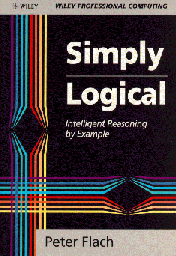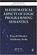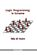Processing ......FreeComputerBooks.com Links to Free Computer, Mathematics, Technical Books all over the World

Simply Logical: Intelligent Reasoning by Example
🌠 Top Free Mathematics Books - 100% Free or Open Source!
• Title: Simply Logical: Intelligent Reasoning by Example
• Author(s) Peter Flach
• Publisher: Wiley; 1 edition (January 15, 1994); eBook (2007)
• Paperback: 240 pages
• eBook: HTML and PDF (247 pages)
• Language: English
• ISBN-10: 0471941522
• ISBN-13: 978-0471941521Book Description

An introduction to Prolog programming for artificial intelligence covering both basic and advanced AI material. A unique advantage to this work is the combination of AI, Prolog and Logic. Each technique is accompanied by a program implementing it. Seeks to simplify the basic concepts of logic programming. Contains exercises and authentic examples to help facilitate the understanding of difficult concepts.

• N/A

Reviews, Ratings, and Recommendations: Related Book Categories: Read and Download Links:Similar Books:
•Introduction to Mathematical Logic (Vilnis Detlovs, et al)

This book explores the principal topics of mathematical logic. It covers propositional logic, first-order logic, first-order number theory, axiomatic set theory, and the theory of computability. Discusses the major results of Gödel, Church, Kleene, Rosser, and Turing.

•A Friendly Introduction to Mathematical Logic (Chris Leary)

In this user-friendly book, readers with no previous study in the field are introduced to the basics of model theory, proof theory, and computability theory, leading to rigorous proofs of Gödel's First and Second Incompleteness Theorems.

•Inductive Logic Programming: Techniques and Applications

This book is an introduction to inductive logic programming (ILP), which aims at a formal framework as well as practical algorithms for inductively learning relational descriptions in the form of logic programs.

•Mathematical Aspects of Logic Programming Semantics

This book discusses applications of Logic Programming to computational logic and potential applications to the integration of models of computation, knowledge representation and reasoning, and the Semantic Web.

•Logic Programming in Scheme (Nils M. Holm)

Questions answered in this little book: What is logic programming? Why is negation hard in logic programming? What is cutting? How do I solve logic puzzles? How is logic programming implemented?

•The Haskell Road to Logic, Maths and Programming (Kees Doets)

The purpose of this book is to teach logic and mathematical reasoning in practice, and to connect logical reasoning with computer programming. Haskell is based on a logical theory of computable functions called the lambda calculus.

•Logic, Programming and Prolog, 2nd Edition (Ulf Nilsson, et al)

This book introduces major new developments in a continually evolving field and includes such topics as concurrency and equational and constraint logic programming.

•An Introduction to Logic Programming Through Prolog (J. Spivey)

This is one of the few texts that combines three essential theses in the study of logic programming: logic, programming, and implementation. The techniques are illustrated by practical examples to explain how logic programming can be implented efficiently.

Book Categories
 :All CategoriesTop Free BooksRecent BooksMiscellaneous BooksComputer EngineeringComputer LanguagesComputer ScienceData Science/DatabasesJava and Java EE (J2EE)Linux and UnixMathematicsMicrosoft and .NETMobile ComputingNetworking and CommunicationsSoftware EngineeringSpecial TopicsWeb Programming
Other Categories